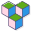CppBuzz# Java program to check if number is positive or negative

``````import java.util.Scanner;

public class Main {

public static void main(String[] args) {
// the number which is greater that 0 is positive
// the number which is less than 0 is negative
// the number which is equal to 0 is 0

Scanner s = new Scanner(System.in);
System.out.println("Enter a number:");
double number = s.nextDouble();
if(number < 0.0) {
System.out.println(number + " is a negative number");
}
else if(number > 0.0) {
System.out.println(number + " is a positive number");
}
else {
System.out.println(number + " is zero");
}
}

}
``````